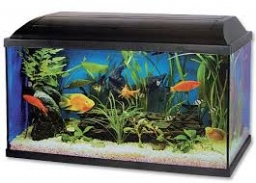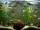# Aquarium

Can 30 liters of water fit in a cuboid aquarium with dimensions a = 3dm b = 6dm c = 5dm?

x =  1

### Step-by-step explanation:Did you find an error or inaccuracy? Feel free to write us. Thank you!Tips to related online calculators
Do you know the volume and unit volume, and want to convert volume units?

#### You need to know the following knowledge to solve this word math problem:

We encourage you to watch this tutorial video on this math problem:

## Related math problems and questions:

• AquariumHow many liters of water can fit in an aquarium measuring 30, 15 and 20 cm?
• Aquarium heightHow high does the water in the aquarium reach, if there are 36 liters of water in it? The length of the aquarium is 60 cm and the width is 4 dm.
• Cuboid aquariumCuboid 25 times 30 cm. How long is third side if cuboid contains 30 liters of water?
• SnailsHow many liters of water will fit in an aquarium with bottom dimensions of 30 cm and 25 cm and a height of 60 cm, if we pour water up to a height of 58 cm? How many most snails can we keep in an aquarium, if we know that snails need 600 cm ^ 3 of water fo
• Cubes into cuboidHow many 12 centimeter cubes fit into the block (cuboid) with 6dm, 8.4dm, and 4.8?
• Swimming poolThe swimming pool has the shape of a block with dimensions of 70dm, 25m, 200cm. How many hl of water can fit into the pool?
• AquariumTry to estimate the weight of the water in an aquarium 50cm long 30cm wide, when poured to a height of 25cm. Calculates the weight of the aquarium's water.
• Water in aquariumThe aquarium cuboid shape with a length of 25 cm and a width of 30 cm is 9 liters of water. Calculate the areas which are wetted with water.
• Empty aquariumHow much does an empty aquarium weigh with dimensions: length = 40 cm, width = 30 cm, height = 20 cm, if 1 dm2 of glass weighs 300 g? Calculate its weight in kilograms.
• Stones in aquariumIn an aquarium with a length 2 m; width 1.5 m and a depth of 2.5 m is a water level up to three-quarters of the depth. Can we place stones with a volume of 2 m3 into the aquarium without water being poured out?
• PebbleThe aquarium with internal dimensions of the bottom 40 cm × 35 cm and a height of 30 cm is filled with two-thirds of water. Calculate how many millimeters the water level in the aquarium rises by dipping a pebble-shaped sphere with a diameter of 18 cm.
• AquariumFind how many dm2 of glass we need to make a block-shaped aquarium (the top is not covered) if the dimensions of the aquarium are to be: width 50 cm, length 120 cm, and height 8.5 dm.
• The cuboidThe cuboid is full to the brim with water. The external dimensions are 95 cm, 120 cm, and 60 cm. The thickness of all walls and the bottom is 5 cm. How many liters of water fit into the cuboid?
• RainfallHow many liters of water did fell in a 32m long and 8m wide garden, if 8mm of rain fell?
• Water levelHow high is the water in the swimming pool with dimensions of 37m in length and 15m in width, if an inlet valve is opened for 10 hours flowing 12 liters of water per second?
• Milk cartonsHow much paper do we need for 12 tetra packs with dimensions 6 cm, 11 cm, and 20 cm? Will 1 liter of milk fit in the box?
• Paper boxCalculate whether 11 dm² of paper is sufficient for gluing a box without a lid with bottom dimensions of 2 dm and 15 cm and 12 cm high. Write result as: 0 = No, 1 = Yes